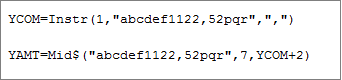# Extracting a variable length amount from a file line

By | February 27, 2019

There might be a situation where we would need to read a file and extract a variable length amount from a line. So, consider an example string/file line- “abcdef1122,52pqr”.

Here the amount which we need to extract is “1122,52”.

Refer the below code snippet-Here,

First, we find the position of “,” and store it in a variable YCOM.

Then, we extract the amount from its starting position which in this case is 7 to the postion of “,” plus the number of decimals there are going to be which in this case is 2, therefore YCOM+2.

Hope this blog helps!